Technical Information Site of Power Supply Design

2016.10.20 Transfer Function

# What are transfer functions? - Frequency Characteristics of Transfer Functions

DC/DC Converters: Sharing of Transfer Functions among Control Systems

Frequency characteristics of transfer functions

Here we consider transfer functions from the standpoint of "Frequency Characteristics of transfer functions". The explanations of "Kirchhoff's Laws and Impedance" in the previous section are closely related, and so should be read in conjunction with this section.

First, consider Fig. 6. This is a simple closed circuit consisting of a resistor and a capacitor. We begin by determining the transfer function for this circuit.

In order to aid visualization, the circuit diagram of Fig. 6 is rewritten as in Fig. 7. Of course, the two circuits are the same. From Fig. 7 we immediately see that ΔVout is the result of dividing ΔVin by the impedances of R and C.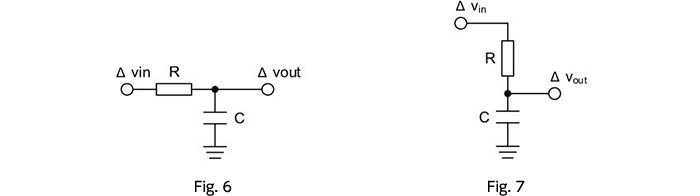Expressed as an equation, this is ΔVout = ΔVin × (C/R+C)), but we use an impedance representation. As explained in the previous section on "Kirchhoff's Laws and Impedance", the impedance of R is represented by R, but for C we have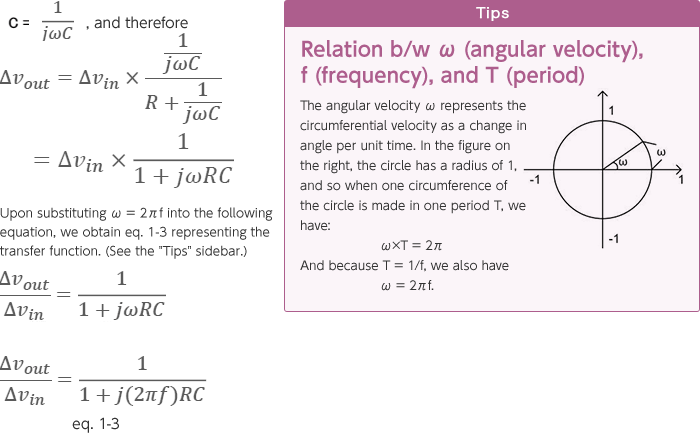Next, we try drawing a Bode diagram. A Bode diagram represents the frequency (f) along the horizontal axis and the gain and phase along the vertical axis, and so the gain and phase must be determined. We begin by finding the gain.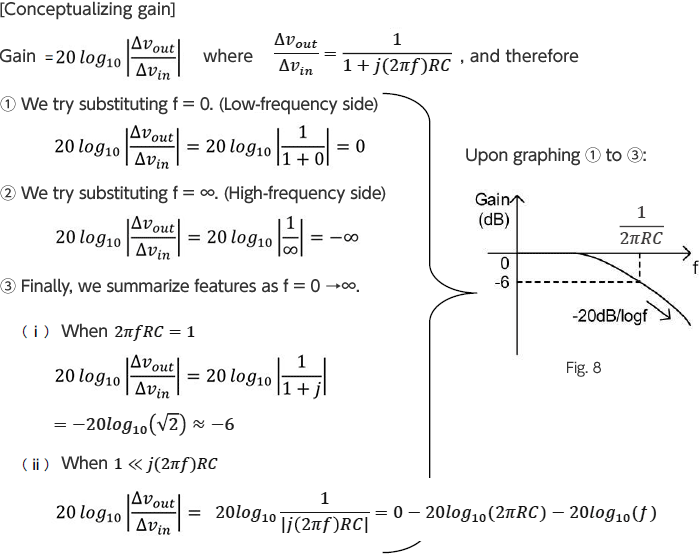Next, we determine the phase.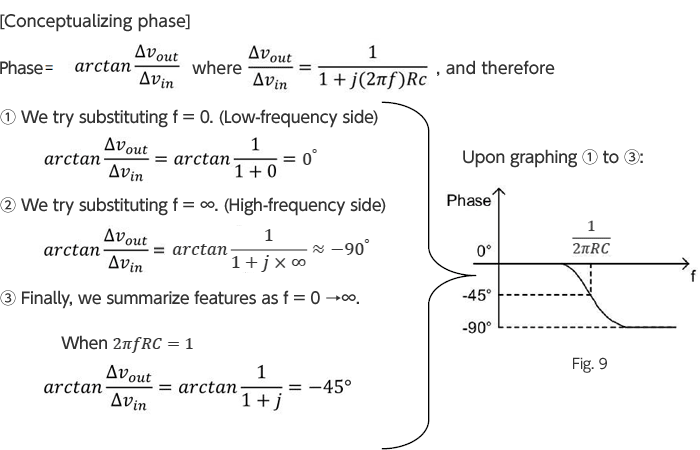The above is summarized in Fig. 10 below. From this the behavior of the gain and phase characteristics should be clear.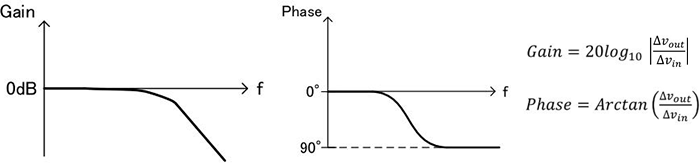Fig. 10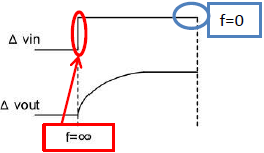Fig. 11

In the previous section on "Kirchhoff's Laws and Impedance", it was stated that the impedance of a capacitor is written "1/jωC. Let's try to understand this in terms of transfer functions using Fig. 11.

Fig. 11 illustrates the step response characteristic of the circuit of Fig. 6. At the moment of a fluctuation in the power supply (equivalent to f = ∞), the capacitor impedance becomes zero, and ΔVout = 0. After a certain time has passed (equivalent to f = 0), it becomes equal to ΔVin.

This can be illustrated as follows, describing the impedance 1/jωC for the step response of the capacitor.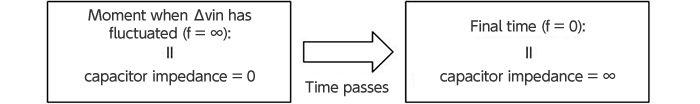Fig. 12

Finally, Fig. 13 gives the impedance representations for elements including a coil as well, and the circuit equivalents for the components when ω = 0 and when ω = ∞, while Fig. 14 describes their frequency characteristics.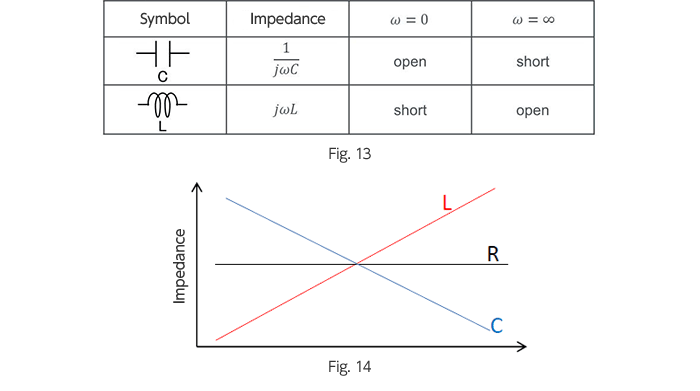This website uses cookies.

By continuing to browse this website without changing your web-browser cookie settings, you are agreeing to our use of cookies.